# Basic Structures¶

## DataType¶

Template “traits” class for other OpenCV primitive data types

template<typename _Tp> class DataType
{
// value_type is always a synonym for _Tp.
typedef _Tp value_type;

// intermediate type used for operations on _Tp.
// it is int for uchar, signed char, unsigned short, signed short and int,
// float for float, double for double, ...
typedef <...> work_type;
// in the case of multi-channel data it is the data type of each channel
typedef <...> channel_type;
enum
{
// CV_8U ... CV_64F
// 1 ...
channels = <...>,
// '1u', '4i', '3f', '2d' etc.
fmt=<...>,
// CV_8UC3, CV_32FC2 ...
type = CV_MAKETYPE(depth, channels)
};
};


The template class DataType is descriptive class for OpenCV primitive data types and other types that comply with the following definition. A primitive OpenCV data type is one of unsigned char, bool, signed char, unsigned short, signed short, int, float, double or a tuple of values of one of these types, where all the values in the tuple have the same type. If you are familiar with OpenCV CvMat ‘s type notation, CV _ 8U ... CV _ 32FC3, CV _ 64FC2 etc., then a primitive type can be defined as a type for which you can give a unique identifier in a form CV_<bit-depth>{U|S|F}C<number_of_channels> . A universal OpenCV structure able to store a single instance of such primitive data type is Vec . Multiple instances of such a type can be stored to a std::vector , Mat , Mat_ , SparseMat , SparseMat_ or any other container that is able to store Vec instances.

The class DataType is basically used to provide some description of such primitive data types without adding any fields or methods to the corresponding classes (and it is actually impossible to add anything to primitive C/C++ data types). This technique is known in C++ as class traits. It’s not DataType itself that is used, but its specialized versions, such as:

template<> class DataType<uchar>
{
typedef uchar value_type;
typedef int work_type;
typedef uchar channel_type;
enum { channel_type = CV_8U, channels = 1, fmt='u', type = CV_8U };
};
...
template<typename _Tp> DataType<std::complex<_Tp> >
{
typedef std::complex<_Tp> value_type;
typedef std::complex<_Tp> work_type;
typedef _Tp channel_type;
// DataDepth is another helper trait class
enum { depth = DataDepth<_Tp>::value, channels=2,
type=CV_MAKETYPE(depth, channels) };
};
...


The main purpose of the classes is to convert compile-time type information to OpenCV-compatible data type identifier, for example:

// allocates 30x40 floating-point matrix
Mat A(30, 40, DataType<float>::type);

Mat B = Mat_<std::complex<double> >(3, 3);
// the statement below will print 6, 2 /* i.e. depth == CV_64F, channels == 2 */
cout << B.depth() << ", " << B.channels() << endl;


that is, such traits are used to tell OpenCV which data type you are working with, even if such a type is not native to OpenCV (the matrix B intialization above compiles because OpenCV defines the proper specialized template class DataType<complex<_Tp> > ). Also, this mechanism is useful (and used in OpenCV this way) for generic algorithms implementations.

## Point_¶

Template class for 2D points

template<typename _Tp> class Point_
{
public:
typedef _Tp value_type;

Point_();
Point_(_Tp _x, _Tp _y);
Point_(const Point_& pt);
Point_(const CvPoint& pt);
Point_(const CvPoint2D32f& pt);
Point_(const Size_<_Tp>& sz);
Point_(const Vec<_Tp, 2>& v);
Point_& operator = (const Point_& pt);
template<typename _Tp2> operator Point_<_Tp2>() const;
operator CvPoint() const;
operator CvPoint2D32f() const;
operator Vec<_Tp, 2>() const;

// computes dot-product (this->x*pt.x + this->y*pt.y)
_Tp dot(const Point_& pt) const;
// computes dot-product using double-precision arithmetics
double ddot(const Point_& pt) const;
// returns true if the point is inside the rectangle "r".
bool inside(const Rect_<_Tp>& r) const;

_Tp x, y;
};


The class represents a 2D point, specified by its coordinatesand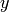. Instance of the class is interchangeable with C structures CvPoint and CvPoint2D32f . There is also cast operator to convert point coordinates to the specified type. The conversion from floating-point coordinates to integer coordinates is done by rounding; in general case the conversion uses operation on each of the coordinates. Besides the class members listed in the declaration above, the following operations on points are implemented:

pt1 = pt2 + pt3;
pt1 = pt2 - pt3;
pt1 = pt2 * a;
pt1 = a * pt2;
pt1 += pt2;
pt1 -= pt2;
pt1 *= a;
double value = norm(pt); // L2 norm
pt1 == pt2;
pt1 != pt2;


For user convenience, the following type aliases are defined:

typedef Point_<int> Point2i;
typedef Point2i Point;
typedef Point_<float> Point2f;
typedef Point_<double> Point2d;


Here is a short example:

Point2f a(0.3f, 0.f), b(0.f, 0.4f);
Point pt = (a + b)*10.f;
cout << pt.x << ", " << pt.y << endl;


## Point3_¶

Template class for 3D points

template<typename _Tp> class Point3_
{
public:
typedef _Tp value_type;

Point3_();
Point3_(_Tp _x, _Tp _y, _Tp _z);
Point3_(const Point3_& pt);
explicit Point3_(const Point_<_Tp>& pt);
Point3_(const CvPoint3D32f& pt);
Point3_(const Vec<_Tp, 3>& v);
Point3_& operator = (const Point3_& pt);
template<typename _Tp2> operator Point3_<_Tp2>() const;
operator CvPoint3D32f() const;
operator Vec<_Tp, 3>() const;

_Tp dot(const Point3_& pt) const;
double ddot(const Point3_& pt) const;

_Tp x, y, z;
};


The class represents a 3D point, specified by its coordinates,and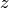. Instance of the class is interchangeable with C structure CvPoint2D32f . Similarly to Point_ , the 3D points’ coordinates can be converted to another type, and the vector arithmetic and comparison operations are also supported.

The following type aliases are available:

typedef Point3_<int> Point3i;
typedef Point3_<float> Point3f;
typedef Point3_<double> Point3d;


## Size_¶

Template class for specfying image or rectangle size.

template<typename _Tp> class Size_
{
public:
typedef _Tp value_type;

Size_();
Size_(_Tp _width, _Tp _height);
Size_(const Size_& sz);
Size_(const CvSize& sz);
Size_(const CvSize2D32f& sz);
Size_(const Point_<_Tp>& pt);
Size_& operator = (const Size_& sz);
_Tp area() const;

operator Size_<int>() const;
operator Size_<float>() const;
operator Size_<double>() const;
operator CvSize() const;
operator CvSize2D32f() const;

_Tp width, height;
};


The class Size_ is similar to Point_ , except that the two members are called width and height instead of x and y . The structure can be converted to and from the old OpenCV structures CvSize and CvSize2D32f . The same set of arithmetic and comparison operations as for Point_ is available.

OpenCV defines the following type aliases:

typedef Size_<int> Size2i;
typedef Size2i Size;
typedef Size_<float> Size2f;


## Rect_¶

Template class for 2D rectangles

template<typename _Tp> class Rect_
{
public:
typedef _Tp value_type;

Rect_();
Rect_(_Tp _x, _Tp _y, _Tp _width, _Tp _height);
Rect_(const Rect_& r);
Rect_(const CvRect& r);
// (x, y) <- org, (width, height) <- sz
Rect_(const Point_<_Tp>& org, const Size_<_Tp>& sz);
// (x, y) <- min(pt1, pt2), (width, height) <- max(pt1, pt2) - (x, y)
Rect_(const Point_<_Tp>& pt1, const Point_<_Tp>& pt2);
Rect_& operator = ( const Rect_& r );
// returns Point_<_Tp>(x, y)
Point_<_Tp> tl() const;
// returns Point_<_Tp>(x+width, y+height)
Point_<_Tp> br() const;

// returns Size_<_Tp>(width, height)
Size_<_Tp> size() const;
// returns width*height
_Tp area() const;

operator Rect_<int>() const;
operator Rect_<float>() const;
operator Rect_<double>() const;
operator CvRect() const;

// x <= pt.x && pt.x < x + width &&
// y <= pt.y && pt.y < y + height ? true : false
bool contains(const Point_<_Tp>& pt) const;

_Tp x, y, width, height;
};


The rectangle is described by the coordinates of the top-left corner (which is the default interpretation of Rect_::x and Rect_::y in OpenCV; though, in your algorithms you may count x and y from the bottom-left corner), the rectangle width and height.

Another assumption OpenCV usually makes is that the top and left boundary of the rectangle are inclusive, while the right and bottom boundaries are not, for example, the method Rect_::contains returns true if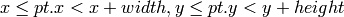And virtually every loop over an image ROI in OpenCV (where ROI is specified by Rect_<int> ) is implemented as:

for(int y = roi.y; y < roi.y + rect.height; y++)
for(int x = roi.x; x < roi.x + rect.width; x++)
{
// ...
}


In addition to the class members, the following operations on rectangles are implemented:

•(shifting rectangle by a certain offset)
•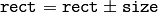(expanding or shrinking rectangle by a certain amount)
• rect += point, rect -= point, rect += size, rect -= size (augmenting operations)
• rect = rect1 & rect2 (rectangle intersection)
• rect = rect1 | rect2 (minimum area rectangle containing rect2 and rect3 )
• rect &= rect1, rect |= rect1 (and the corresponding augmenting operations)
• rect == rect1, rect != rect1 (rectangle comparison)

Example. Here is how the partial ordering on rectangles can be established (rect1rect2):

template<typename _Tp> inline bool
operator <= (const Rect_<_Tp>& r1, const Rect_<_Tp>& r2)
{
return (r1 & r2) == r1;
}


For user convenience, the following type alias is available:

typedef Rect_<int> Rect;


## RotatedRect¶

Possibly rotated rectangle

class RotatedRect
{
public:
// constructors
RotatedRect();
RotatedRect(const Point2f& _center, const Size2f& _size, float _angle);
RotatedRect(const CvBox2D& box);

// returns minimal up-right rectangle that contains the rotated rectangle
Rect boundingRect() const;
// backward conversion to CvBox2D
operator CvBox2D() const;

// mass center of the rectangle
Point2f center;
// size
Size2f size;
// rotation angle in degrees
float angle;
};


The class RotatedRect replaces the old CvBox2D and fully compatible with it.

## TermCriteria¶

Termination criteria for iterative algorithms

class TermCriteria
{
public:
enum { COUNT=1, MAX_ITER=COUNT, EPS=2 };

// constructors
TermCriteria();
// type can be MAX_ITER, EPS or MAX_ITER+EPS.
// type = MAX_ITER means that only the number of iterations does matter;
// type = EPS means that only the required precision (epsilon) does matter
//    (though, most algorithms put some limit on the number of iterations anyway)
// type = MAX_ITER + EPS means that algorithm stops when
// either the specified number of iterations is made,
// or when the specified accuracy is achieved - whatever happens first.
TermCriteria(int _type, int _maxCount, double _epsilon);
TermCriteria(const CvTermCriteria& criteria);
operator CvTermCriteria() const;

int type;
int maxCount;
double epsilon;
};


The class TermCriteria replaces the old CvTermCriteria and fully compatible with it.

## Matx¶

Template class for small matrices

template<typename T, int m, int n> class Matx
{
public:
typedef T value_type;
enum { depth = DataDepth<T>::value, channels = m*n,
type = CV_MAKETYPE(depth, channels) };

// various methods
...

Tp val[m*n];
};

typedef Matx<float, 1, 2> Matx12f;
typedef Matx<double, 1, 2> Matx12d;
...
typedef Matx<float, 1, 6> Matx16f;
typedef Matx<double, 1, 6> Matx16d;

typedef Matx<float, 2, 1> Matx21f;
typedef Matx<double, 2, 1> Matx21d;
...
typedef Matx<float, 6, 1> Matx61f;
typedef Matx<double, 6, 1> Matx61d;

typedef Matx<float, 2, 2> Matx22f;
typedef Matx<double, 2, 2> Matx22d;
...
typedef Matx<float, 6, 6> Matx66f;
typedef Matx<double, 6, 6> Matx66d;


The class represents small matrices, which type and size are known at compile time. If you need more flexible type, use Mat . The elements of a matrix M are accessible using M(i,j) notation, and most of the common matrix operations (see also MatrixExpressions ) are available. If you need to do some operation on Matx that is not implemented, it is easy to convert the matrix to Mat and backwards.

Matx33f m(1, 2, 3,
4, 5, 6,
7, 8, 9);
cout << sum(Mat(m*m.t())) << endl;


## Vec¶

Template class for short numerical vectors

template<typename T, int cn> class Vec : public Matx<T, cn, 1>
{
public:
typedef T value_type;
enum { depth = DataDepth<T>::value, channels = cn,
type = CV_MAKETYPE(depth, channels) };

// various methods ...
};

typedef Vec<uchar, 2> Vec2b;
typedef Vec<uchar, 3> Vec3b;
typedef Vec<uchar, 4> Vec4b;

typedef Vec<short, 2> Vec2s;
typedef Vec<short, 3> Vec3s;
typedef Vec<short, 4> Vec4s;

typedef Vec<int, 2> Vec2i;
typedef Vec<int, 3> Vec3i;
typedef Vec<int, 4> Vec4i;

typedef Vec<float, 2> Vec2f;
typedef Vec<float, 3> Vec3f;
typedef Vec<float, 4> Vec4f;
typedef Vec<float, 6> Vec6f;

typedef Vec<double, 2> Vec2d;
typedef Vec<double, 3> Vec3d;
typedef Vec<double, 4> Vec4d;
typedef Vec<double, 6> Vec6d;


Vec is a partial case of Matx . It is possible to convert Vec<T,2> to/from Point_ , Vec<T,3> to/from Point3_ , and Vec<T,4> to CvScalar or Scalar . The elements of Vec are accessed using operator[] . All the expected vector operations are implemented too:

•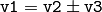,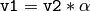,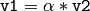(plus the corresponding augmenting operations; note that these operations apply to the each computed vector component)
• v1 == v2, v1 != v2
• norm(v1) (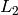-norm)

The class Vec is commonly used to describe pixel types of multi-channel arrays, see Mat_ description.

## Scalar_¶

4-element vector

template<typename _Tp> class Scalar_ : public Vec<_Tp, 4>
{
public:
Scalar_();
Scalar_(_Tp v0, _Tp v1, _Tp v2=0, _Tp v3=0);
Scalar_(const CvScalar& s);
Scalar_(_Tp v0);
static Scalar_<_Tp> all(_Tp v0);
operator CvScalar() const;

template<typename T2> operator Scalar_<T2>() const;

Scalar_<_Tp> mul(const Scalar_<_Tp>& t, double scale=1 ) const;
template<typename T2> void convertTo(T2* buf, int channels, int unroll_to=0) const;
};

typedef Scalar_<double> Scalar;


The template class Scalar_ and it’s double-precision instantiation Scalar represent 4-element vector. Being derived from Vec<_Tp, 4> , they can be used as typical 4-element vectors, but in addition they can be converted to/from CvScalar . The type Scalar is widely used in OpenCV for passing pixel values and it is a drop-in replacement for CvScalar that was used for the same purpose in the earlier versions of OpenCV.

## Range¶

Specifies a continuous subsequence (a.k.a. slice) of a sequence.

class Range
{
public:
Range();
Range(int _start, int _end);
Range(const CvSlice& slice);
int size() const;
bool empty() const;
static Range all();
operator CvSlice() const;

int start, end;
};


The class is used to specify a row or column span in a matrix ( Mat ), and for many other purposes. Range(a,b) is basically the same as a:b in Matlab or a..b in Python. As in Python, start is inclusive left boundary of the range, and end is exclusive right boundary of the range. Such a half-opened interval is usually denoted as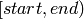.

The static method Range::all() returns some special variable that means “the whole sequence” or “the whole range”, just like ” : ” in Matlab or ” ... ” in Python. All the methods and functions in OpenCV that take Range support this special Range::all() value, but of course, in the case of your own custom processing you will probably have to check and handle it explicitly:

void my_function(..., const Range& r, ....)
{
if(r == Range::all()) {
// process all the data
}
else {
// process [r.start, r.end)
}
}


## Ptr¶

A template class for smart reference-counting pointers

template<typename _Tp> class Ptr
{
public:
// default constructor
Ptr();
// constructor that wraps the object pointer
Ptr(_Tp* _obj);
// destructor: calls release()
~Ptr();
// copy constructor; increments ptr's reference counter
Ptr(const Ptr& ptr);
// assignment operator; decrements own reference counter
// (with release()) and increments ptr's reference counter
Ptr& operator = (const Ptr& ptr);
// increments reference counter
// decrements reference counter; when it becomes 0,
// delete_obj() is called
void release();
// user-specified custom object deletion operation.
// by default, "delete obj;" is called
void delete_obj();
// returns true if obj == 0;
bool empty() const;

_Tp* operator -> ();
const _Tp* operator -> () const;

// return the underlying object pointer;
// thanks to the methods, the Ptr<_Tp> can be
operator _Tp* ();
operator const _Tp*() const;
protected:
// the encapsulated object pointer
_Tp* obj;
// the associated reference counter
int* refcount;
};


The class Ptr<_Tp> is a template class that wraps pointers of the corresponding type. It is similar to shared_ptr that is a part of Boost library ( http://www.boost.org/doc/libs/1_40_0/libs/smart_ptr/shared_ptr.htm ) and also a part of the C++0x standard.

By using this class you can get the following capabilities:

• default constructor, copy constructor and assignment operator for an arbitrary C++ class or a C structure. For some objects, like files, windows, mutexes, sockets etc, copy constructor or assignment operator are difficult to define. For some other objects, like complex classifiers in OpenCV, copy constructors are absent and not easy to implement. Finally, some of complex OpenCV and your own data structures may have been written in C. However, copy constructors and default constructors can simplify programming a lot; besides, they are often required (e.g. by STL containers). By wrapping a pointer to such a complex object TObj to Ptr<TObj> you will automatically get all of the necessary constructors and the assignment operator.
• all the above-mentioned operations running very fast, regardless of the data size, i.e. as “O(1)” operations. Indeed, while some structures, like std::vector provide a copy constructor and an assignment operator, the operations may take considerable time if the data structures are big. But if the structures are put into Ptr<> , the overhead becomes small and independent of the data size.
• automatic destruction, even for C structures. See the example below with FILE* .
• heterogeneous collections of objects. The standard STL and most other C++ and OpenCV containers can only store objects of the same type and the same size. The classical solution to store objects of different types in the same container is to store pointers to the base class base_class_t* instead, but when you loose the automatic memory management. Again, by using Ptr<base_class_t>() instead of the raw pointers, you can solve the problem.

The class Ptr treats the wrapped object as a black box, the reference counter is allocated and managed separately. The only thing the pointer class needs to know about the object is how to deallocate it. This knowledge is incapsulated in Ptr::delete_obj() method, which is called when the reference counter becomes 0. If the object is a C++ class instance, no additional coding is needed, because the default implementation of this method calls delete obj; . However, if the object is deallocated in a different way, then the specialized method should be created. For example, if you want to wrap FILE , the delete_obj may be implemented as following:

template<> inline void Ptr<FILE>::delete_obj()
{
fclose(obj); // no need to clear the pointer afterwards,
// it is done externally.
}
...

// now use it:
Ptr<FILE> f(fopen("myfile.txt", "r"));
if(f.empty())
throw ...;
fprintf(f, ....);
...
// the file will be closed automatically by the Ptr<FILE> destructor.


Note : The reference increment/decrement operations are implemented as atomic operations, and therefore it is normally safe to use the classes in multi-threaded applications. The same is true for Mat and other C++ OpenCV classes that operate on the reference counters.

## Mat¶

OpenCV C++ n-dimensional dense array class.

class CV_EXPORTS Mat
{
public:
// ... a lot of methods ...
...

/*! includes several bit-fields:
- the magic signature
- continuity flag
- depth
- number of channels
*/
int flags;
//! the array dimensionality, >= 2
int dims;
//! the number of rows and columns or (-1, -1) when the array has more than 2 dimensions
int rows, cols;
//! pointer to the data
uchar* data;

//! pointer to the reference counter;
// when array points to user-allocated data, the pointer is NULL
int* refcount;

// other members
...
};


The class Mat represents an n-dimensional dense numerical single-channel or multi-channel array. It can be used to store real or complex-valued vectors and matrices, grayscale or color images, voxel volumes, vector fields, point clouds, tensors, histograms (though, very high-dimensional histograms may be better stored in a SparseMat ). The data layout of array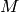is defined by the array M.step[] , so that the address of element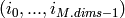, whereis computed as: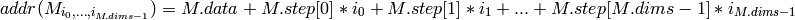In the case of 2-dimensional array the above formula is reduced to: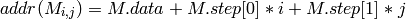Note that M.step[i] >= M.step[i+1] (in fact, M.step[i] >= M.step[i+1]*M.size[i+1] ), that is, 2-dimensional matrices are stored row-by-row, 3-dimensional matrices are stored plane-by-plane etc. M.step[M.dims-1] is minimal and always equal to the element size M.elemSize() .

That is, the data layout in Mat is fully compatible with CvMat , IplImage and CvMatND types from OpenCV 1.x, as well as with majority of dense array types from the standard toolkits and SDKs, such as Numpy (ndarray), Win32 (independent device bitmaps) etc, i.e. any other array that uses “steps”, a.k.a. “strides”, to compute position of a pixel. Because of such compatibility, it is possible to make a Mat header for user-allocated data and process it in-place using OpenCV functions.

There are many different ways to create Mat object. Here are the some popular ones:

• using create(nrows, ncols, type) method or

the similar constructor

Mat(nrows, ncols, type[, fillValue]) constructor.

A new array of the specified size and specifed type will be allocated.

type has the same meaning as in cvCreateMat() method,

e.g.

CV_8UC1 means 8-bit single-channel array,

CV_32FC2 means 2-channel (i.e. complex) floating-point array etc:

// make 7x7 complex matrix filled with 1+3j.
cv::Mat M(7,7,CV_32FC2,Scalar(1,3));
// and now turn M to 100x60 15-channel 8-bit matrix.
// The old content will be deallocated
M.create(100,60,CV_8UC(15));


As noted in the introduction of this chapter, create() will only allocate a new array when the current array shape

or type are different from the specified.

• similarly to above, you can create a multi-dimensional array:

// create 100x100x100 8-bit array
int sz[] = {100, 100, 100};
cv::Mat bigCube(3, sz, CV_8U, Scalar::all(0));


note that it is pass number of dimensions =1 to the Mat constructor, but the created array will be 2-dimensional, with the number of columns set to 1. That’s why Mat::dims is always >= 2 (can also be 0 when the array is empty)

• by using a copy constructor or assignment operator, where on the right side it can

be a array or expression, see below. Again, as noted in the introduction, array assignment is O(1) operation because it only copies the header and increases the reference counter.

Mat::clone() method can be used to get a full

(a.k.a. deep) copy of the array when you need it.

• by constructing a header for a part of another array. It can be a single row, single column,

several rows, several columns, rectangular region in the array (called a minor in algebra) or a diagonal. Such operations are also O(1), because the new header will reference the same data. You can actually modify a part of the array using this feature, e.g.

// add 5-th row, multiplied by 3 to the 3rd row
M.row(3) = M.row(3) + M.row(5)*3;

// now copy 7-th column to the 1-st column
// M.col(1) = M.col(7); // this will not work
Mat M1 = M.col(1);
M.col(7).copyTo(M1);

// create new 320x240 image
cv::Mat img(Size(320,240),CV_8UC3);
// select a roi
cv::Mat roi(img, Rect(10,10,100,100));
// fill the ROI with (0,255,0) (which is green in RGB space);
// the original 320x240 image will be modified
roi = Scalar(0,255,0);


Thanks to the additional datastart and dataend members, it is possible to

compute the relative sub-array position in the main

“container” array using locateROI() :

Mat A = Mat::eye(10, 10, CV_32S);
// extracts A columns, 1 (inclusive) to 3 (exclusive).
Mat B = A(Range::all(), Range(1, 3));
// extracts B rows, 5 (inclusive) to 9 (exclusive).
// that is, C ~ A(Range(5, 9), Range(1, 3))
Mat C = B(Range(5, 9), Range::all());
Size size; Point ofs;
C.locateROI(size, ofs);
// size will be (width=10,height=10) and the ofs will be (x=1, y=5)


As in the case of whole matrices, if you need a deep copy, use clone() method

of the extracted sub-matrices.

• by making a header for user-allocated-data. It can be useful for

1. processing “foreign” data using OpenCV (e.g. when you implement

a DirectShow filter or a processing module for gstreamer etc.), e.g.

void process_video_frame(const unsigned char* pixels,
int width, int height, int step)
{
cv::Mat img(height, width, CV_8UC3, pixels, step);
cv::GaussianBlur(img, img, cv::Size(7,7), 1.5, 1.5);
}

2. for quick initialization of small matrices and/or super-fast element access

double m = {{a, b, c}, {d, e, f}, {g, h, i}};
cv::Mat M = cv::Mat(3, 3, CV_64F, m).inv();

partial yet very common cases of this “user-allocated data” case are conversions

from

CvMat and IplImage to Mat . For this purpose there are special constructors

taking pointers to

CvMat or IplImage and the optional

flag indicating whether to copy the data or not.

Backward conversion from

Mat to CvMat or IplImage is provided via cast operators

Mat::operator CvMat() const an Mat::operator IplImage() .

The operators do

not copy the data.

IplImage* img = cvLoadImage("greatwave.jpg", 1);
Mat mtx(img); // convert IplImage* -> cv::Mat
CvMat oldmat = mtx; // convert cv::Mat -> CvMat
CV_Assert(oldmat.cols == img->width && oldmat.rows == img->height &&
oldmat.data.ptr == (uchar*)img->imageData && oldmat.step == img->widthStep);

• by using MATLAB-style array initializers, zeros(), ones(), eye() , e.g.:

// create a double-precision identity martix and add it to M.
M += Mat::eye(M.rows, M.cols, CV_64F);

• by using comma-separated initializer:

// create 3x3 double-precision identity matrix
Mat M = (Mat_<double>(3,3) << 1, 0, 0, 0, 1, 0, 0, 0, 1);


here we first call constructor of Mat_ class (that we describe further) with the proper parameters, and then we just put << operator followed by comma-separated values that can be constants, variables, expressions etc. Also, note the extra parentheses that are needed to avoid compiler errors.

Once array is created, it will be automatically managed by using reference-counting mechanism (unless the array header is built on top of user-allocated data, in which case you should handle the data by yourself). The array data will be deallocated when no one points to it; if you want to release the data pointed by a array header before the array destructor is called, use Mat::release() .

The next important thing to learn about the array class is element access. Earlier it was shown how to compute address of each array element. Normally, it’s not needed to use the formula directly in your code. If you know the array element type (which can be retrieved using the method Mat::type() ), you can access element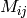of 2-dimensional array as:

M.at<double>(i,j) += 1.f;


assuming that M is double-precision floating-point array. There are several variants of the method at for different number of dimensions.

If you need to process a whole row of a 2d array, the most efficient way is to get the pointer to the row first, and then just use plain C operator [] :

// compute sum of positive matrix elements
// (assuming that M is double-precision matrix)
double sum=0;
for(int i = 0; i < M.rows; i++)
{
const double* Mi = M.ptr<double>(i);
for(int j = 0; j < M.cols; j++)
sum += std::max(Mi[j], 0.);
}


Some operations, like the above one, do not actually depend on the array shape, they just process elements of an array one by one (or elements from multiple arrays that have the same coordinates, e.g. array addition). Such operations are called element-wise and it makes sense to check whether all the input/output arrays are continuous, i.e. have no gaps in the end of each row, and if yes, process them as a single long row:

// compute sum of positive matrix elements, optimized variant
double sum=0;
int cols = M.cols, rows = M.rows;
if(M.isContinuous())
{
cols *= rows;
rows = 1;
}
for(int i = 0; i < rows; i++)
{
const double* Mi = M.ptr<double>(i);
for(int j = 0; j < cols; j++)
sum += std::max(Mi[j], 0.);
}


in the case of continuous matrix the outer loop body will be executed just once, so the overhead will be smaller, which will be especially noticeable in the case of small matrices.

Finally, there are STL-style iterators that are smart enough to skip gaps between successive rows:

// compute sum of positive matrix elements, iterator-based variant
double sum=0;
MatConstIterator_<double> it = M.begin<double>(), it_end = M.end<double>();
for(; it != it_end; ++it)
sum += std::max(*it, 0.);


The matrix iterators are random-access iterators, so they can be passed to any STL algorithm, including std::sort() .

## Matrix Expressions¶

This is a list of implemented matrix operations that can be combined in arbitrary complex expressions (here A , B stand for matrices ( Mat ), s for a scalar ( Scalar ),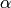for a real-valued scalar ( double )):

• addition, subtraction, negation: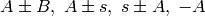• scaling:,• per-element multiplication and division:• matrix multiplication: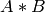• transposition: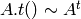• matrix inversion and pseudo-inversion, solving linear systems and least-squares problems: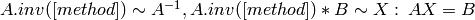• comparison: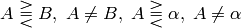.

The result of comparison is 8-bit single channel mask, which elements are set to 255 (if the particular element or pair of elements satisfy the condition) and 0 otherwise.

• bitwise logical operations: A & B, A & s, A | B, A | s, A textasciicircum B, A textasciicircum s, ~ A

• element-wise minimum and maximum:• element-wise absolute value:• cross-product, dot-product: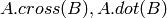• any function of matrix or matrices and scalars that returns a matrix or a scalar, such as

norm() , mean() , sum() , countNonZero() , trace() ,

determinant() , repeat() etc.

• matrix initializers ( eye(), zeros(), ones() ), matrix comma-separated initializers,

matrix constructors and operators that extract sub-matrices (see

Mat description).

• verb “Mat_<destination_type>()” constructors to cast the result to the proper type.

Note, however, that comma-separated initializers and probably some other operations may require additional explicit Mat() or verb “Mat_<T>()” constuctor calls to resolve possible ambiguity.

Below is the formal description of the Mat methods.

## cv::Mat::Mat¶

(1) Mat::Mat()
(2) Mat::Mat(int rows, int cols, int type)
(3) Mat::Mat(Size size, int type)
(4) Mat::Mat(int rows, int cols, int type, const Scalar& s)
(5) Mat::Mat(Size size, int type, const Scalar& s)
(6) Mat::Mat(const Mat& m)
(7) Mat::Mat(int rows, int cols, int type, void* data, size_t step=AUTO_STEP)
(8) Mat::Mat(Size size, int type, void* data, size_t step=AUTO_STEP)
(9) Mat::Mat(const Mat& m, const Range& rowRange, const Range& colRange)
(10) Mat::Mat(const Mat& m, const Rect& roi)
(11) Mat::Mat(const CvMat* m, bool copyData=false)
(12) Mat::Mat(const IplImage* img, bool copyData=false)
(13) template<typename T, int n> explicit Mat::Mat(const Vec<T, n>& vec, bool copyData=true)
(14) template<typename T, int m, int n> explicit Mat::Mat(const Matx<T, m, n>& vec, bool copyData=true)
(15) template<typename T> explicit Mat::Mat(const vector<T>& vec, bool copyData=false)
(16) Mat::Mat(const MatExpr& expr)
(17) Mat::Mat(int ndims, const int* sizes, int type)
(18) Mat::Mat(int ndims, const int* sizes, int type, const Scalar& s)
(19) Mat::Mat(int ndims, const int* sizes, int type, void* data, const size_t* steps=0)
(20) Mat::Mat(const Mat& m, const Range* ranges)

Various array constructors

Parameters: ndims – The array dimensionality rows – The number of rows in 2D array cols – The number of columns in 2D array size – The 2D array size: Size(cols, rows) . Note that in the Size() constructor the number of rows and the number of columns go in the reverse order. sizes – The array of integers, specifying the n-dimensional array shape type – The array type, use CV_8UC1, ..., CV_64FC4 to create 1-4 channel matrices, or CV_8UC(n), ..., CV_64FC(n) to create multi-channel (up to CV_MAX_CN channels) matrices s – The optional value to initialize each matrix element with. To set all the matrix elements to the particular value after the construction, use the assignment operator Mat::operator=(const Scalar& value) . data – Pointer to the user data. Matrix constructors that take data and step parameters do not allocate matrix data. Instead, they just initialize the matrix header that points to the specified data, i.e. no data is copied. This operation is very efficient and can be used to process external data using OpenCV functions. The external data is not automatically deallocated, user should take care of it. step – The data buddy. This optional parameter specifies the number of bytes that each matrix row occupies. The value should include the padding bytes in the end of each row, if any. If the parameter is missing (set to cv::AUTO_STEP ), no padding is assumed and the actual step is calculated as cols*elemSize() , see Mat::elemSize (). steps – The array of ndims-1 steps in the case of multi-dimensional array (the last step is always set to the element size). If not specified, the matrix is assumed to be continuous. m – The array that (in whole, a partly) is assigned to the constructed matrix. No data is copied by these constructors. Instead, the header pointing to m data, or its sub-array, is constructed and the associated with it reference counter, if any, is incremented. That is, when you modify the matrix formed using such a constructor, you will also modify the corresponding elements of m . If you want to have an independent copy of the sub-array, use Mat::clone() . img – Pointer to the old-style IplImage image structure. By default, the data is shared between the original image and the new matrix, but when copyData is set, the full copy of the image data is created. vec – STL vector, which elements will form the matrix. The matrix will have a single column and the number of rows equal to the number of vector elements. Type of the matrix will match the type of vector elements. The constructor can handle arbitrary types, for which there is properly declared DataType , i.e. the vector elements must be primitive numbers or uni-type numerical tuples of numbers. Mixed-type structures are not supported, of course. Note that the corresponding constructor is explicit, meaning that STL vectors are not automatically converted to Mat instances, you should write Mat(vec) explicitly. Another obvious note: unless you copied the data into the matrix ( copyData=true ), no new elements should be added to the vector, because it can potentially yield vector data reallocation, and thus the matrix data pointer will become invalid. copyData – Specifies, whether the underlying data of the STL vector, or the old-style CvMat or IplImage should be copied to (true) or shared with (false) the newly constructed matrix. When the data is copied, the allocated buffer will be managed using Mat ‘s reference counting mechanism. While when the data is shared, the reference counter will be NULL, and you should not deallocate the data until the matrix is not destructed. rowRange – The range of the m ‘s rows to take. As usual, the range start is inclusive and the range end is exclusive. Use Range::all() to take all the rows. colRange – The range of the m ‘s columns to take. Use Range::all() to take all the columns. ranges – The array of selected ranges of m along each dimensionality

.

Parameters: expr – Matrix expression. See Matrix Expressions .

These are various constructors that form a matrix. As noticed in the , often the default constructor is enough, and the proper matrix will be allocated by an OpenCV function. The constructed matrix can further be assigned to another matrix or matrix expression, in which case the old content is dereferenced, or be allocated with Mat::create .

## cv::Mat::Mat¶

Mat::textasciitilde Mat()

Matrix destructor

The matrix destructor calls Mat::release .

## cv::Mat::operator =¶

Mat& Mat::operator = (const Mat& m)
Mat& Mat::operator = (const MatExpr_Base& expr)
Mat& operator = (const Scalar& s)

Matrix assignment operators

Parameters: m – The assigned, right-hand-side matrix. Matrix assignment is O(1) operation, that is, no data is copied. Instead, the data is shared and the reference counter, if any, is incremented. Before assigning new data, the old data is dereferenced via Mat::release . expr – The assigned matrix expression object. As opposite to the first form of assignment operation, the second form can reuse already allocated matrix if it has the right size and type to fit the matrix expression result. It is automatically handled by the real function that the matrix expressions is expanded to. For example, C=A+B is expanded to cv::add(A, B, C) , and add() will take care of automatic C reallocation. s – The scalar, assigned to each matrix element. The matrix size or type is not changed.

These are the available assignment operators, and they all are very different, so, please, look at the operator parameters description.

## cv::Mat::operator MatExpr¶

Mat::operator MatExpr_<Mat, Mat>() const

Mat-to-MatExpr cast operator

The cast operator should not be called explicitly. It is used internally by the Matrix Expressions engine.

## cv::Mat::row¶

Mat Mat::row(int i) const

Makes a matrix header for the specified matrix row

Parameters: i – the 0-based row index

The method makes a new header for the specified matrix row and returns it. This is O(1) operation, regardless of the matrix size. The underlying data of the new matrix will be shared with the original matrix. Here is the example of one of the classical basic matrix processing operations, axpy, used by LU and many other algorithms:

inline void matrix_axpy(Mat& A, int i, int j, double alpha)
{
A.row(i) += A.row(j)*alpha;
}


Important note . In the current implementation the following code will not work as expected:

Mat A;
...
A.row(i) = A.row(j); // will not work


This is because A.row(i) forms a temporary header, which is further assigned another header. Remember, each of these operations is O(1), i.e. no data is copied. Thus, the above assignment will have absolutely no effect, while you may have expected j-th row being copied to i-th row. To achieve that, you should either turn this simple assignment into an expression, or use Mat::copyTo method:

Mat A;
...
// works, but looks a bit obscure.
A.row(i) = A.row(j) + 0;

// this is a bit longer, but the recommended method.
Mat Ai = A.row(i); M.row(j).copyTo(Ai);


## cv::Mat::col¶

Mat Mat::col(int j) const

Makes a matrix header for the specified matrix column

Parameters: j – the 0-based column index

The method makes a new header for the specified matrix column and returns it. This is O(1) operation, regardless of the matrix size. The underlying data of the new matrix will be shared with the original matrix. See also Mat::row description.

## cv::Mat::rowRange¶

Mat Mat::rowRange(int startrow, int endrow) const
Mat Mat::rowRange(const Range& r) const

Makes a matrix header for the specified row span

Parameters: startrow – the 0-based start index of the row span endrow – the 0-based ending index of the row span r – The Range() structure containing both the start and the end indices

The method makes a new header for the specified row span of the matrix. Similarly to Mat::row() and Mat::col() , this is O(1) operation.

## cv::Mat::colRange¶

Mat Mat::colRange(int startcol, int endcol) const
Mat Mat::colRange(const Range& r) const

Makes a matrix header for the specified row span

Parameters: startcol – the 0-based start index of the column span endcol – the 0-based ending index of the column span r – The Range() structure containing both the start and the end indices

The method makes a new header for the specified column span of the matrix. Similarly to Mat::row() and Mat::col() , this is O(1) operation.

## cv::Mat::diag¶

Mat Mat::diag(int d) const static Mat Mat::diag(const Mat& matD)

Extracts diagonal from a matrix, or creates a diagonal matrix.

Parameters: d – index of the diagonal, with the following meaning: d=0 the main diagonal d>0 a diagonal from the lower half, e.g. d=1 means the diagonal immediately below the main one d<0 a diagonal from the upper half, e.g. d=1 means the diagonal immediately above the main one matD – single-column matrix that will form the diagonal matrix.

The method makes a new header for the specified matrix diagonal. The new matrix will be represented as a single-column matrix. Similarly to Mat::row() and Mat::col() , this is O(1) operation.

## cv::Mat::clone¶

Mat Mat::clone() const

Creates full copy of the array and the underlying data.

The method creates full copy of the array. The original step[] are not taken into the account. That is, the array copy will be a continuous array occupying total()*elemSize() bytes.

## cv::Mat::copyTo¶

void Mat::copyTo(Mat& m ) const void Mat::copyTo( Mat& m, const Mat& mask) const

Copies the matrix to another one.

Parameters: m – The destination matrix. If it does not have a proper size or type before the operation, it will be reallocated mask – The operation mask. Its non-zero elements indicate, which matrix elements need to be copied

The method copies the matrix data to another matrix. Before copying the data, the method invokes

m.create(this->size(), this->type);


so that the destination matrix is reallocated if needed. While m.copyTo(m); will work as expected, i.e. will have no effect, the function does not handle the case of a partial overlap between the source and the destination matrices.

When the operation mask is specified, and the Mat::create call shown above reallocated the matrix, the newly allocated matrix is initialized with all 0’s before copying the data.

## cv::Mat::convertTo¶

void Mat::convertTo(Mat& m, int rtype, double alpha=1, double beta=0) const

Converts array to another datatype with optional scaling.

Parameters: m – The destination matrix. If it does not have a proper size or type before the operation, it will be reallocated rtype – The desired destination matrix type, or rather, the depth (since the number of channels will be the same with the source one). If rtype is negative, the destination matrix will have the same type as the source. alpha – The optional scale factor beta – The optional delta, added to the scaled values.

The method converts source pixel values to the target datatype. saturate_cast<> is applied in the end to avoid possible overflows: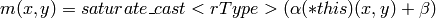## cv::Mat::assignTo¶

void Mat::assignTo(Mat& m, int type=-1) const

Functional form of convertTo

Parameters: m – The destination array type – The desired destination array depth (or -1 if it should be the same as the source one).

This is internal-use method called by the Matrix Expressions engine.

## cv::Mat::setTo¶

Mat& Mat::setTo(const Scalar& s, const Mat& mask=Mat())

Sets all or some of the array elements to the specified value.

Parameters: s – Assigned scalar, which is converted to the actual array type mask – The operation mask of the same size as *this

This is the advanced variant of Mat::operator=(const Scalar& s) operator.

## cv::Mat::reshape¶

Mat Mat::reshape(int cn, int rows=0) const

Changes the 2D matrix’s shape and/or the number of channels without copying the data.

Parameters: cn – The new number of channels. If the parameter is 0, the number of channels remains the same. rows – The new number of rows. If the parameter is 0, the number of rows remains the same.

The method makes a new matrix header for *this elements. The new matrix may have different size and/or different number of channels. Any combination is possible, as long as:

1. No extra elements is included into the new matrix and no elements are excluded. Consequently,

the product

rows*cols*channels() must stay the same after the transformation.

2. No data is copied, i.e. this is O(1) operation. Consequently, if you change the number of rows, or the operation changes elements’ row indices in some other way, the matrix must be continuous. See Mat::isContinuous() .

Here is some small example. Assuming, there is a set of 3D points that are stored as STL vector, and you want to represent the points as 3xN matrix. Here is how it can be done:

std::vector<cv::Point3f> vec;
...

Mat pointMat = Mat(vec). // convert vector to Mat, O(1) operation
reshape(1). // make Nx3 1-channel matrix out of Nx1 3-channel.
// Also, an O(1) operation
t(); // finally, transpose the Nx3 matrix.
// This involves copying of all the elements


## cv::Mat::t¶

MatExpr Mat::t() const

Transposes the matrix

The method performs matrix transposition by means of matrix expressions. It does not perform the actual transposition, but returns a temporary “matrix transposition” object that can be further used as a part of more complex matrix expression or be assigned to a matrix:

Mat A1 = A + Mat::eye(A.size(), A.type)*lambda;
Mat C = A1.t()*A1; // compute (A + lambda*I)^t * (A + lamda*I)


## cv::Mat::inv¶

MatExpr Mat::inv(int method=DECOMP_LU) const

Inverses the matrix

Parameters: method – The matrix inversion method, one of DECOMP_LU LU decomposition. The matrix must be non-singular DECOMP_CHOLESKY Cholesky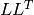decomposition, for symmetrical positively defined matrices only. About twice faster than LU on big matrices. DECOMP_SVD SVD decomposition. The matrix can be a singular or even non-square, then the pseudo-inverse is computed

The method performs matrix inversion by means of matrix expressions, i.e. a temporary “matrix inversion” object is returned by the method, and can further be used as a part of more complex matrix expression or be assigned to a matrix.

## cv::Mat::mul¶

MatExpr Mat::mul(const Mat& m, double scale=1) const
MatExpr Mat::mul(const MatExpr& m, double scale=1) const

Performs element-wise multiplication or division of the two matrices

Parameters: m – Another matrix, of the same type and the same size as *this , or a matrix expression scale – The optional scale factor

The method returns a temporary object encoding per-element array multiplication, with optional scale. Note that this is not a matrix multiplication, which corresponds to a simpler “*” operator.

Here is a example:

Mat C = A.mul(5/B); // equivalent to divide(A, B, C, 5)


## cv::Mat::cross¶

Mat Mat::cross(const Mat& m) const

Computes cross-product of two 3-element vectors

Parameters: m – Another cross-product operand

The method computes cross-product of the two 3-element vectors. The vectors must be 3-elements floating-point vectors of the same shape and the same size. The result will be another 3-element vector of the same shape and the same type as operands.

## cv::Mat::dot¶

double Mat::dot(const Mat& m) const

Computes dot-product of two vectors

Parameters: m – Another dot-product operand.

The method computes dot-product of the two matrices. If the matrices are not single-column or single-row vectors, the top-to-bottom left-to-right scan ordering is used to treat them as 1D vectors. The vectors must have the same size and the same type. If the matrices have more than one channel, the dot products from all the channels are summed together.

## cv::Mat::zeros¶

static MatExpr Mat::zeros(int rows, int cols, int type) static MatExpr Mat::zeros(Size size, int type) static MatExpr Mat::zeros(int ndims, const int* sizes, int type)

Returns zero array of the specified size and type

Parameters: ndims – The array dimensionality rows – The number of rows cols – The number of columns size – Alternative matrix size specification: Size(cols, rows) sizes – The array of integers, specifying the array shape type – The created matrix type

The method returns Matlab-style zero array initializer. It can be used to quickly form a constant array and use it as a function parameter, as a part of matrix expression, or as a matrix initializer.

Mat A;
A = Mat::zeros(3, 3, CV_32F);


Note that in the above sample a new matrix will be allocated only if A is not 3x3 floating-point matrix, otherwise the existing matrix A will be filled with 0’s.

## cv::Mat::ones¶

static MatExpr Mat::ones(int rows, int cols, int type) static MatExpr Mat::ones(Size size, int type) static MatExpr Mat::ones(int ndims, const int* sizes, int type)

Returns array of all 1’s of the specified size and type

Parameters: ndims – The array dimensionality rows – The number of rows cols – The number of columns size – Alternative matrix size specification: Size(cols, rows) sizes – The array of integers, specifying the array shape type – The created matrix type

The method returns Matlab-style ones’ array initializer, similarly to Mat::zeros() . Note that using this method you can initialize an array with arbitrary value, using the following Matlab idiom:

Mat A = Mat::ones(100, 100, CV_8U)*3; // make 100x100 matrix filled with 3.


The above operation will not form 100x100 matrix of ones and then multiply it by 3. Instead, it will just remember the scale factor (3 in this case) and use it when actually invoking the matrix initializer.

## cv::Mat::eye¶

static MatExpr Mat::eye(int rows, int cols, int type) static MatExpr Mat::eye(Size size, int type)

Returns identity matrix of the specified size and type

Parameters: rows – The number of rows cols – The number of columns size – Alternative matrix size specification: Size(cols, rows) type – The created matrix type

The method returns Matlab-style identity matrix initializer, similarly to Mat::zeros() . Similarly to Mat::ones , you can use a scale operation to create a scaled identity matrix efficiently:

// make a 4x4 diagonal matrix with 0.1's on the diagonal.
Mat A = Mat::eye(4, 4, CV_32F)*0.1;


## cv::Mat::create¶

void Mat::create(int rows, int cols, int type) void Mat::create(Size size, int type) void Mat::create(int ndims, const int* sizes, int type)

Allocates new array data if needed.

Parameters: ndims – The new array dimensionality rows – The new number of rows cols – The new number of columns size – Alternative new matrix size specification: Size(cols, rows) sizes – The array of integers, specifying the new array shape type – The new matrix type

This is one of the key Mat methods. Most new-style OpenCV functions and methods that produce arrays call this method for each output array. The method uses the following algorithm:

1. if the current array shape and the type match the new ones, return immediately.
2. otherwise, dereference the previous data by calling Mat::release()
4. allocate the new data of total()*elemSize() bytes
5. allocate the new, associated with the data, reference counter and set it to 1.

Such a scheme makes the memory management robust and efficient at the same time, and also saves quite a bit of typing for the user, i.e. usually there is no need to explicitly allocate output arrays. That is, instead of writing:

Mat color;
...
Mat gray(color.rows, color.cols, color.depth());
cvtColor(color, gray, CV_BGR2GRAY);


you can simply write:

Mat color;
...
Mat gray;
cvtColor(color, gray, CV_BGR2GRAY);


because cvtColor , as well as most of OpenCV functions, calls Mat::create() for the output array internally.

Increments the reference counter

The method increments the reference counter, associated with the matrix data. If the matrix header points to an external data (see Mat::Mat() ), the reference counter is NULL, and the method has no effect in this case. Normally, the method should not be called explicitly, to avoid memory leaks. It is called implicitly by the matrix assignment operator. The reference counter increment is the atomic operation on the platforms that support it, thus it is safe to operate on the same matrices asynchronously in different threads.

## cv::Mat::release¶

void Mat::release()

Decrements the reference counter and deallocates the matrix if needed

The method decrements the reference counter, associated with the matrix data. When the reference counter reaches 0, the matrix data is deallocated and the data and the reference counter pointers are set to NULL’s. If the matrix header points to an external data (see Mat::Mat() ), the reference counter is NULL, and the method has no effect in this case.

This method can be called manually to force the matrix data deallocation. But since this method is automatically called in the destructor, or by any other method that changes the data pointer, it is usually not needed. The reference counter decrement and check for 0 is the atomic operation on the platforms that support it, thus it is safe to operate on the same matrices asynchronously in different threads.

## cv::Mat::resize¶

void Mat::resize(size_t sz) const

Changes the number of matrix rows

Parameters: sz – The new number of rows

The method changes the number of matrix rows. If the matrix is reallocated, the first min(Mat::rows, sz) rows are preserved. The method emulates the corresponding method of STL vector class.

## Mat::push_back¶

template<typename T> void Mat::push_back(const T& elem) template<typename T> void Mat::push_back(const Mat_<T>& elem)

Adds elements to the bottom of the matrix

Parameters: elem – The added element(s).

The methods add one or more elements to the bottom of the matrix. They emulate the corresponding method of STL vector class. When elem is Mat , its type and the number of columns must be the same as in the container matrix.

## Mat::pop_back¶

template<typename T> void Mat::pop_back(size_t nelems=1)

Removes elements from the bottom of the matrix.

Parameters: nelems – The number of rows removed. If it is greater than the total number of rows, an exception is thrown.

The method removes one or more rows from the bottom of the matrix.

## cv::Mat::locateROI¶

void Mat::locateROI(Size& wholeSize, Point& ofs) const

Locates matrix header within a parent matrix

Parameters: wholeSize – The output parameter that will contain size of the whole matrix, which *this is a part of. ofs – The output parameter that will contain offset of *this inside the whole matrix

After you extracted a submatrix from a matrix using Mat::row() , Mat::col() , Mat::rowRange() , Mat::colRange() etc., the result submatrix will point just to the part of the original big matrix. However, each submatrix contains some information (represented by datastart and dataend fields), using which it is possible to reconstruct the original matrix size and the position of the extracted submatrix within the original matrix. The method locateROI does exactly that.

Mat& Mat::adjustROI(int dtop, int dbottom, int dleft, int dright)

Adjust submatrix size and position within the parent matrix

Parameters: dtop – The shift of the top submatrix boundary upwards dbottom – The shift of the bottom submatrix boundary downwards dleft – The shift of the left submatrix boundary to the left dright – The shift of the right submatrix boundary to the right

The method is complimentary to the Mat::locateROI() . Indeed, the typical use of these functions is to determine the submatrix position within the parent matrix and then shift the position somehow. Typically it can be needed for filtering operations, when pixels outside of the ROI should be taken into account. When all the method’s parameters are positive, it means that the ROI needs to grow in all directions by the specified amount, i.e.

A.adjustROI(2, 2, 2, 2);


increases the matrix size by 4 elements in each direction and shifts it by 2 elements to the left and 2 elements up, which brings in all the necessary pixels for the filtering with 5x5 kernel.

It’s user responsibility to make sure that adjustROI does not cross the parent matrix boundary. If it does, the function will signal an error.

The function is used internally by the OpenCV filtering functions, like filter2D() , morphological operations etc.

## cv::Mat::operator()¶

Mat Mat::operator()( Range rowRange, Range colRange) const
Mat Mat::operator()( const Rect& roi ) const Mat Mat::operator()( const Ranges* ranges) const

Extracts a rectangular submatrix

Parameters: rowRange – The start and the end row of the extracted submatrix. The upper boundary is not included. To select all the rows, use Range::all() colRange – The start and the end column of the extracted submatrix. The upper boundary is not included. To select all the columns, use Range::all() roi – The extracted submatrix specified as a rectangle ranges – The array of selected ranges along each array dimension

The operators make a new header for the specified sub-array of *this . They are the most generalized forms of Mat::row() , Mat::col() , Mat::rowRange() and Mat::colRange() . For example, A(Range(0, 10), Range::all()) is equivalent to A.rowRange(0, 10) . Similarly to all of the above, the operators are O(1) operations, i.e. no matrix data is copied.

## cv::Mat::operator CvMat¶

Mat::operator CvMat() const

Creates CvMat header for the matrix

The operator makes CvMat header for the matrix without copying the underlying data. The reference counter is not taken into account by this operation, thus you should make sure than the original matrix is not deallocated while the CvMat header is used. The operator is useful for intermixing the new and the old OpenCV API’s, e.g:

Mat img(Size(320, 240), CV_8UC3);
...

CvMat cvimg = img;
mycvOldFunc( &cvimg, ...);


where mycvOldFunc is some function written to work with OpenCV 1.x data structures.

## cv::Mat::operator IplImage¶

Mat::operator IplImage() const

Creates IplImage header for the matrix

The operator makes IplImage header for the matrix without copying the underlying data. You should make sure than the original matrix is not deallocated while the IplImage header is used. Similarly to Mat::operator CvMat , the operator is useful for intermixing the new and the old OpenCV API’s.

## cv::Mat::total¶

size_t Mat::total() const

Returns the total number of array elements.

The method returns the number of array elements (e.g. number of pixels if the array represents an image).

## cv::Mat::isContinuous¶

bool Mat::isContinuous() const

Reports whether the matrix is continuous or not

The method returns true if the matrix elements are stored continuously, i.e. without gaps in the end of each row, and false otherwise. Obviously, 1x1 or 1xN matrices are always continuous. Matrices created with Mat::create() are always continuous, but if you extract a part of the matrix using Mat::col() , Mat::diag() etc. or constructed a matrix header for externally allocated data, such matrices may no longer have this property.

The continuity flag is stored as a bit in Mat::flags field, and is computed automatically when you construct a matrix header, thus the continuity check is very fast operation, though it could be, in theory, done as following:

// alternative implementation of Mat::isContinuous()
bool myCheckMatContinuity(const Mat& m)
{
//return (m.flags & Mat::CONTINUOUS_FLAG) != 0;
return m.rows == 1 || m.step == m.cols*m.elemSize();
}


The method is used in a quite a few of OpenCV functions, and you are welcome to use it as well. The point is that element-wise operations (such as arithmetic and logical operations, math functions, alpha blending, color space transformations etc.) do not depend on the image geometry, and thus, if all the input and all the output arrays are continuous, the functions can process them as very long single-row vectors. Here is the example of how alpha-blending function can be implemented.

template<typename T>
void alphaBlendRGBA(const Mat& src1, const Mat& src2, Mat& dst)
{
const float alpha_scale = (float)std::numeric_limits<T>::max(),
inv_scale = 1.f/alpha_scale;

CV_Assert( src1.type() == src2.type() &&
src1.type() == CV_MAKETYPE(DataType<T>::depth, 4) &&
src1.size() == src2.size());
Size size = src1.size();
dst.create(size, src1.type());

// here is the idiom: check the arrays for continuity and,
// if this is the case,
// treat the arrays as 1D vectors
if( src1.isContinuous() && src2.isContinuous() && dst.isContinuous() )
{
size.width *= size.height;
size.height = 1;
}
size.width *= 4;

for( int i = 0; i < size.height; i++ )
{
// when the arrays are continuous,
// the outer loop is executed only once
const T* ptr1 = src1.ptr<T>(i);
const T* ptr2 = src2.ptr<T>(i);
T* dptr = dst.ptr<T>(i);

for( int j = 0; j < size.width; j += 4 )
{
float alpha = ptr1[j+3]*inv_scale, beta = ptr2[j+3]*inv_scale;
dptr[j] = saturate_cast<T>(ptr1[j]*alpha + ptr2[j]*beta);
dptr[j+1] = saturate_cast<T>(ptr1[j+1]*alpha + ptr2[j+1]*beta);
dptr[j+2] = saturate_cast<T>(ptr1[j+2]*alpha + ptr2[j+2]*beta);
dptr[j+3] = saturate_cast<T>((1 - (1-alpha)*(1-beta))*alpha_scale);
}
}
}


This trick, while being very simple, can boost performance of a simple element-operation by 10-20 percents, especially if the image is rather small and the operation is quite simple.

Also, note that we use another OpenCV idiom in this function - we call Mat::create() for the destination array instead of checking that it already has the proper size and type. And while the newly allocated arrays are always continuous, we still check the destination array, because create() does not always allocate a new matrix.

## cv::Mat::elemSize¶

size_t Mat::elemSize() const

Returns matrix element size in bytes

The method returns the matrix element size in bytes. For example, if the matrix type is CV_16SC3 , the method will return 3*sizeof(short) or 6.

## cv::Mat::elemSize1¶

size_t Mat::elemSize1() const

Returns size of each matrix element channel in bytes

The method returns the matrix element channel size in bytes, that is, it ignores the number of channels. For example, if the matrix type is CV_16SC3 , the method will return sizeof(short) or 2.

## cv::Mat::type¶

int Mat::type() const

Returns matrix element type

The method returns the matrix element type, an id, compatible with the CvMat type system, like CV_16SC3 or 16-bit signed 3-channel array etc.

## cv::Mat::depth¶

int Mat::depth() const

Returns matrix element depth

The method returns the matrix element depth id, i.e. the type of each individual channel. For example, for 16-bit signed 3-channel array the method will return CV_16S . The complete list of matrix types:

• CV_8U - 8-bit unsigned integers ( 0..255 )
• CV_8S - 8-bit signed integers ( -128..127 )
• CV_16U - 16-bit unsigned integers ( 0..65535 )
• CV_16S - 16-bit signed integers ( -32768..32767 )
• CV_32S - 32-bit signed integers ( -2147483648..2147483647 )
• CV_32F - 32-bit floating-point numbers ( -FLT_MAX..FLT_MAX, INF, NAN )
• CV_64F - 64-bit floating-point numbers ( -DBL_MAX..DBL_MAX, INF, NAN )

## cv::Mat::channels¶

int Mat::channels() const

Returns matrix element depth

The method returns the number of matrix channels.

## cv::Mat::step1¶

size_t Mat::step1() const

Returns normalized step

The method returns the matrix step, divided by Mat::elemSize1() . It can be useful for fast access to arbitrary matrix element.

## cv::Mat::size¶

Size Mat::size() const

Returns the matrix size

The method returns the matrix size: Size(cols, rows) .

## cv::Mat::empty¶

bool Mat::empty() const

Returns true if the array has no elemens

The method returns true if Mat::total() is 0 or if Mat::data is NULL. Because of pop_back() and resize() methods M.total() == 0 does not imply that M.data == NULL .

## cv::Mat::ptr¶

uchar* Mat::ptr(int i=0)
const uchar* Mat::ptr(int i=0) const
template<typename _Tp> _Tp* Mat::ptr(int i=0)
template<typename _Tp> const _Tp* Mat::ptr(int i=0) const

Return pointer to the specified matrix row

Parameters: i – The 0-based row index

The methods return uchar* or typed pointer to the specified matrix row. See the sample in Mat::isContinuous() () on how to use these methods.

## cv::Mat::at¶

template<typename T> T& Mat::at(int i) const
template<typename T> const T& Mat::at(int i) const
template<typename T> T& Mat::at(int i, int j)
template<typename T> const T& Mat::at(int i, int j) const
template<typename T> T& Mat::at(Point pt)
template<typename T> const T& Mat::at(Point pt) const
template<typename T> T& Mat::at(int i, int j, int k)
template<typename T> const T& Mat::at(int i, int j, int k) const
template<typename T> T& Mat::at(const int* idx)
template<typename T> const T& Mat::at(const int* idx) const

Return reference to the specified array element

Parameters: j, k (i,) – Indices along the dimensions 0, 1 and 2, respectively pt – The element position specified as Point(j,i) idx – The array of Mat::dims indices

The template methods return reference to the specified array element. For the sake of higher performance the index range checks are only performed in Debug configuration.

Note that the variants with a single index (i) can be used to access elements of single-row or single-column 2-dimensional arrays. That is, if, for example, A is 1 x N floating-point matrix and B is M x 1 integer matrix, you can simply write A.at<float>(k+4) and B.at<int>(2*i+1) instead of A.at<float>(0,k+4) and B.at<int>(2*i+1,0) , respectively.

Here is an example of initialization of a Hilbert matrix:

Mat H(100, 100, CV_64F);
for(int i = 0; i < H.rows; i++)
for(int j = 0; j < H.cols; j++)
H.at<double>(i,j)=1./(i+j+1);


## cv::Mat::begin¶

template<typename _Tp> MatIterator_<_Tp> Mat::begin() template<typename _Tp> MatConstIterator_<_Tp> Mat::begin() const

Return the matrix iterator, set to the first matrix element

The methods return the matrix read-only or read-write iterators. The use of matrix iterators is very similar to the use of bi-directional STL iterators. Here is the alpha blending function rewritten using the matrix iterators:

template<typename T>
void alphaBlendRGBA(const Mat& src1, const Mat& src2, Mat& dst)
{
typedef Vec<T, 4> VT;

const float alpha_scale = (float)std::numeric_limits<T>::max(),
inv_scale = 1.f/alpha_scale;

CV_Assert( src1.type() == src2.type() &&
src1.type() == DataType<VT>::type &&
src1.size() == src2.size());
Size size = src1.size();
dst.create(size, src1.type());

MatConstIterator_<VT> it1 = src1.begin<VT>(), it1_end = src1.end<VT>();
MatConstIterator_<VT> it2 = src2.begin<VT>();
MatIterator_<VT> dst_it = dst.begin<VT>();

for( ; it1 != it1_end; ++it1, ++it2, ++dst_it )
{
VT pix1 = *it1, pix2 = *it2;
float alpha = pix1*inv_scale, beta = pix2*inv_scale;
*dst_it = VT(saturate_cast<T>(pix1*alpha + pix2*beta),
saturate_cast<T>(pix1*alpha + pix2*beta),
saturate_cast<T>(pix1*alpha + pix2*beta),
saturate_cast<T>((1 - (1-alpha)*(1-beta))*alpha_scale));
}
}


## cv::Mat::end¶

template<typename _Tp> MatIterator_<_Tp> Mat::end() template<typename _Tp> MatConstIterator_<_Tp> Mat::end() const

Return the matrix iterator, set to the after-last matrix element

The methods return the matrix read-only or read-write iterators, set to the point following the last matrix element.

## Mat_¶

Template matrix class derived from Mat

template<typename _Tp> class Mat_ : public Mat
{
public:
// ... some specific methods
//         and
// no new extra fields
};


The class Mat_<_Tp> is a “thin” template wrapper on top of Mat class. It does not have any extra data fields, nor it or Mat have any virtual methods and thus references or pointers to these two classes can be freely converted one to another. But do it with care, e.g.:

// create 100x100 8-bit matrix
Mat M(100,100,CV_8U);
// this will compile fine. no any data conversion will be done.
Mat_<float>& M1 = (Mat_<float>&)M;
// the program will likely crash at the statement below
M1(99,99) = 1.f;


While Mat is sufficient in most cases, Mat_ can be more convenient if you use a lot of element access operations and if you know matrix type at compile time. Note that Mat::at<_Tp>(int y, int x) and Mat_<_Tp>::operator ()(int y, int x) do absolutely the same and run at the same speed, but the latter is certainly shorter:

Mat_<double> M(20,20);
for(int i = 0; i < M.rows; i++)
for(int j = 0; j < M.cols; j++)
M(i,j) = 1./(i+j+1);
Mat E, V;
eigen(M,E,V);
cout << E.at<double>(0,0)/E.at<double>(M.rows-1,0);


How to use Mat_ for multi-channel images/matrices? This is simple - just pass Vec as Mat_ parameter:

// allocate 320x240 color image and fill it with green (in RGB space)
Mat_<Vec3b> img(240, 320, Vec3b(0,255,0));
// now draw a diagonal white line
for(int i = 0; i < 100; i++)
img(i,i)=Vec3b(255,255,255);
// and now scramble the 2nd (red) channel of each pixel
for(int i = 0; i < img.rows; i++)
for(int j = 0; j < img.cols; j++)
img(i,j) ^= (uchar)(i ^ j);


## NAryMatIterator¶

n-ary multi-dimensional array iterator

class CV_EXPORTS NAryMatIterator
{
public:
//! the default constructor
NAryMatIterator();
//! the full constructor taking arbitrary number of n-dim matrices
NAryMatIterator(const Mat** arrays, Mat* planes, int narrays=-1);
//! the separate iterator initialization method
void init(const Mat** arrays, Mat* planes, int narrays=-1);

//! proceeds to the next plane of every iterated matrix
NAryMatIterator& operator ++();
//! proceeds to the next plane of every iterated matrix (postfix increment operator)
NAryMatIterator operator ++(int);

...
int nplanes; // the total number of planes
};


The class is used for implementation of unary, binary and, generally, n-ary element-wise operations on multi-dimensional arrays. Some of the arguments of n-ary function may be continuous arrays, some may be not. It is possible to use conventional MatIterator ‘s for each array, but it can be a big overhead to increment all of the iterators after each small operations. That’s where NAryMatIterator can be used. Using it, you can iterate though several matrices simultaneously as long as they have the same geometry (dimensionality and all the dimension sizes are the same). On each iteration it.planes , it.planes , ... will be the slices of the corresponding matrices.

Here is an example of how you can compute a normalized and thresholded 3D color histogram:

void computeNormalizedColorHist(const Mat& image, Mat& hist, int N, double minProb)
{
const int histSize[] = {N, N, N};

// make sure that the histogram has proper size and type
hist.create(3, histSize, CV_32F);

// and clear it
hist = Scalar(0);

// the loop below assumes that the image
// is 8-bit 3-channel, so let's check it.
CV_Assert(image.type() == CV_8UC3);
MatConstIterator_<Vec3b> it = image.begin<Vec3b>(),
it_end = image.end<Vec3b>();
for( ; it != it_end; ++it )
{
const Vec3b& pix = *it;
hist.at<float>(pix*N/256, pix*N/256, pix*N/256) += 1.f;
}

minProb *= image.rows*image.cols;
Mat plane;
NAryMatIterator it(&hist, &plane, 1);
double s = 0;
// iterate through the matrix. on each iteration
// it.planes[*] (of type Mat) will be set to the current plane.
for(int p = 0; p < it.nplanes; p++, ++it)
{
threshold(it.planes, it.planes, minProb, 0, THRESH_TOZERO);
s += sum(it.planes);
}

s = 1./s;
it = NAryMatIterator(&hist, &plane, 1);
for(int p = 0; p < it.nplanes; p++, ++it)
it.planes *= s;
}


## SparseMat¶

Sparse n-dimensional array.

class SparseMat
{
public:
typedef SparseMatIterator iterator;
typedef SparseMatConstIterator const_iterator;

// internal structure - sparse matrix header
struct Hdr
{
...
};

// sparse matrix node - element of a hash table
struct Node
{
size_t hashval;
size_t next;
int idx[CV_MAX_DIM];
};

////////// constructors and destructor //////////
// default constructor
SparseMat();
// creates matrix of the specified size and type
SparseMat(int dims, const int* _sizes, int _type);
// copy constructor
SparseMat(const SparseMat& m);
// converts dense array to the sparse form,
// if try1d is true and matrix is a single-column matrix (Nx1),
// then the sparse matrix will be 1-dimensional.
SparseMat(const Mat& m, bool try1d=false);
// converts old-style sparse matrix to the new-style.
// all the data is copied, so that "m" can be safely
// deleted after the conversion
SparseMat(const CvSparseMat* m);
// destructor
~SparseMat();

///////// assignment operations ///////////

// this is O(1) operation; no data is copied
SparseMat& operator = (const SparseMat& m);
// (equivalent to the corresponding constructor with try1d=false)
SparseMat& operator = (const Mat& m);

// creates full copy of the matrix
SparseMat clone() const;

// copy all the data to the destination matrix.
// the destination will be reallocated if needed.
void copyTo( SparseMat& m ) const;
// converts 1D or 2D sparse matrix to dense 2D matrix.
// If the sparse matrix is 1D, then the result will
// be a single-column matrix.
void copyTo( Mat& m ) const;
// converts arbitrary sparse matrix to dense matrix.
// multiplies all the matrix elements by the specified scalar
void convertTo( SparseMat& m, int rtype, double alpha=1 ) const;
// converts sparse matrix to dense matrix with optional type conversion and scaling.
// When rtype=-1, the destination element type will be the same
// as the sparse matrix element type.
// Otherwise rtype will specify the depth and
// the number of channels will remain the same is in the sparse matrix
void convertTo( Mat& m, int rtype, double alpha=1, double beta=0 ) const;

// not used now
void assignTo( SparseMat& m, int type=-1 ) const;

// reallocates sparse matrix. If it was already of the proper size and type,
// it is simply cleared with clear(), otherwise,
// the old matrix is released (using release()) and the new one is allocated.
void create(int dims, const int* _sizes, int _type);
// sets all the matrix elements to 0, which means clearing the hash table.
void clear();
// manually increases reference counter to the header.
// decreses the header reference counter, when it reaches 0,
// the header and all the underlying data are deallocated.
void release();

// converts sparse matrix to the old-style representation.
// all the elements are copied.
operator CvSparseMat*() const;
// size of each element in bytes
// (the matrix nodes will be bigger because of
//  element indices and other SparseMat::Node elements).
size_t elemSize() const;
// elemSize()/channels()
size_t elemSize1() const;

// the same is in Mat
int type() const;
int depth() const;
int channels() const;

// returns the array of sizes and 0 if the matrix is not allocated
const int* size() const;
// returns i-th size (or 0)
int size(int i) const;
// returns the matrix dimensionality
int dims() const;
// returns the number of non-zero elements
size_t nzcount() const;

// compute element hash value from the element indices:
// 1D case
size_t hash(int i0) const;
// 2D case
size_t hash(int i0, int i1) const;
// 3D case
size_t hash(int i0, int i1, int i2) const;
// n-D case
size_t hash(const int* idx) const;

// low-level element-acccess functions,
// special variants for 1D, 2D, 3D cases and the generic one for n-D case.
//
// return pointer to the matrix element.
//  if the element is there (it's non-zero), the pointer to it is returned
//  if it's not there and createMissing=false, NULL pointer is returned
//  if it's not there and createMissing=true, then the new element
//    is created and initialized with 0. Pointer to it is returned
//  If the optional hashval pointer is not NULL, the element hash value is
//  not computed, but *hashval is taken instead.
uchar* ptr(int i0, bool createMissing, size_t* hashval=0);
uchar* ptr(int i0, int i1, bool createMissing, size_t* hashval=0);
uchar* ptr(int i0, int i1, int i2, bool createMissing, size_t* hashval=0);
uchar* ptr(const int* idx, bool createMissing, size_t* hashval=0);

// higher-level element access functions:
// ref<_Tp>(i0,...[,hashval]) - equivalent to *(_Tp*)ptr(i0,...true[,hashval]).
//    always return valid reference to the element.
//    If it's did not exist, it is created.
// find<_Tp>(i0,...[,hashval]) - equivalent to (_const Tp*)ptr(i0,...false[,hashval]).
//    return pointer to the element or NULL pointer if the element is not there.
// value<_Tp>(i0,...[,hashval]) - equivalent to
//    { const _Tp* p = find<_Tp>(i0,...[,hashval]); return p ? *p : _Tp(); }
//    that is, 0 is returned when the element is not there.
// note that _Tp must match the actual matrix type -
// the functions do not do any on-fly type conversion

// 1D case
template<typename _Tp> _Tp& ref(int i0, size_t* hashval=0);
template<typename _Tp> _Tp value(int i0, size_t* hashval=0) const;
template<typename _Tp> const _Tp* find(int i0, size_t* hashval=0) const;

// 2D case
template<typename _Tp> _Tp& ref(int i0, int i1, size_t* hashval=0);
template<typename _Tp> _Tp value(int i0, int i1, size_t* hashval=0) const;
template<typename _Tp> const _Tp* find(int i0, int i1, size_t* hashval=0) const;

// 3D case
template<typename _Tp> _Tp& ref(int i0, int i1, int i2, size_t* hashval=0);
template<typename _Tp> _Tp value(int i0, int i1, int i2, size_t* hashval=0) const;
template<typename _Tp> const _Tp* find(int i0, int i1, int i2, size_t* hashval=0) const;

// n-D case
template<typename _Tp> _Tp& ref(const int* idx, size_t* hashval=0);
template<typename _Tp> _Tp value(const int* idx, size_t* hashval=0) const;
template<typename _Tp> const _Tp* find(const int* idx, size_t* hashval=0) const;

// erase the specified matrix element.
// When there is no such element, the methods do nothing
void erase(int i0, int i1, size_t* hashval=0);
void erase(int i0, int i1, int i2, size_t* hashval=0);
void erase(const int* idx, size_t* hashval=0);

// return the matrix iterators,
//   pointing to the first sparse matrix element,
SparseMatIterator begin();
SparseMatConstIterator begin() const;
//   ... or to the point after the last sparse matrix element
SparseMatIterator end();
SparseMatConstIterator end() const;

// and the template forms of the above methods.
// _Tp must match the actual matrix type.
template<typename _Tp> SparseMatIterator_<_Tp> begin();
template<typename _Tp> SparseMatConstIterator_<_Tp> begin() const;
template<typename _Tp> SparseMatIterator_<_Tp> end();
template<typename _Tp> SparseMatConstIterator_<_Tp> end() const;

// return value stored in the sparse martix node
template<typename _Tp> _Tp& value(Node* n);
template<typename _Tp> const _Tp& value(const Node* n) const;

////////////// some internal-use methods ///////////////
...

// pointer to the sparse matrix header
Hdr* hdr;
};


The class SparseMat represents multi-dimensional sparse numerical arrays. Such a sparse array can store elements of any type that Mat can store. “Sparse” means that only non-zero elements are stored (though, as a result of operations on a sparse matrix, some of its stored elements can actually become 0. It’s up to the user to detect such elements and delete them using SparseMat::erase ). The non-zero elements are stored in a hash table that grows when it’s filled enough, so that the search time is O(1) in average (regardless of whether element is there or not). Elements can be accessed using the following methods:

1. query operations ( SparseMat::ptr and the higher-level SparseMat::ref , SparseMat::value and SparseMat::find ), e.g.:

const int dims = 5;
int size[] = {10, 10, 10, 10, 10};
SparseMat sparse_mat(dims, size, CV_32F);
for(int i = 0; i < 1000; i++)
{
int idx[dims];
for(int k = 0; k < dims; k++)
idx[k] = rand()
sparse_mat.ref<float>(idx) += 1.f;
}

2. sparse matrix iterators. Like Mat iterators and unlike MatND iterators, the sparse matrix iterators are STL-style, that is, the iteration loop is familiar to C++ users:

// prints elements of a sparse floating-point matrix
// and the sum of elements.
SparseMatConstIterator_<float>
it = sparse_mat.begin<float>(),
it_end = sparse_mat.end<float>();
double s = 0;
int dims = sparse_mat.dims();
for(; it != it_end; ++it)
{
// print element indices and the element value
const Node* n = it.node();
printf("(")
for(int i = 0; i < dims; i++)
printf("
printf(":
s += *it;
}
printf("Element sum is


If you run this loop, you will notice that elements are enumerated in no any logical order (lexicographical etc.), they come in the same order as they stored in the hash table, i.e. semi-randomly. You may collect pointers to the nodes and sort them to get the proper ordering. Note, however, that pointers to the nodes may become invalid when you add more elements to the matrix; this is because of possible buffer reallocation.

3. a combination of the above 2 methods when you need to process 2 or more sparse matrices simultaneously, e.g. this is how you can compute unnormalized cross-correlation of the 2 floating-point sparse matrices:

double cross_corr(const SparseMat& a, const SparseMat& b)
{
const SparseMat *_a = &a, *_b = &b;
// if b contains less elements than a,
// it's faster to iterate through b
if(_a->nzcount() > _b->nzcount())
std::swap(_a, _b);
SparseMatConstIterator_<float> it = _a->begin<float>(),
it_end = _a->end<float>();
double ccorr = 0;
for(; it != it_end; ++it)
{
// take the next element from the first matrix
float avalue = *it;
const Node* anode = it.node();
// and try to find element with the same index in the second matrix.
// since the hash value depends only on the element index,
// we reuse hashvalue stored in the node
float bvalue = _b->value<float>(anode->idx,&anode->hashval);
ccorr += avalue*bvalue;
}
return ccorr;
}


## SparseMat_¶

Template sparse n-dimensional array class derived from SparseMat

template<typename _Tp> class SparseMat_ : public SparseMat
{
public:
typedef SparseMatIterator_<_Tp> iterator;
typedef SparseMatConstIterator_<_Tp> const_iterator;

// constructors;
// the created matrix will have data type = DataType<_Tp>::type
SparseMat_();
SparseMat_(int dims, const int* _sizes);
SparseMat_(const SparseMat& m);
SparseMat_(const SparseMat_& m);
SparseMat_(const Mat& m);
SparseMat_(const CvSparseMat* m);
// assignment operators; data type conversion is done when necessary
SparseMat_& operator = (const SparseMat& m);
SparseMat_& operator = (const SparseMat_& m);
SparseMat_& operator = (const Mat& m);
SparseMat_& operator = (const MatND& m);

// equivalent to the correspoding parent class methods
SparseMat_ clone() const;
void create(int dims, const int* _sizes);
operator CvSparseMat*() const;

// overriden methods that do extra checks for the data type
int type() const;
int depth() const;
int channels() const;

// more convenient element access operations.
// ref() is retained (but <_Tp> specification is not need anymore);
// operator () is equivalent to SparseMat::value<_Tp>
_Tp& ref(int i0, size_t* hashval=0);
_Tp operator()(int i0, size_t* hashval=0) const;
_Tp& ref(int i0, int i1, size_t* hashval=0);
_Tp operator()(int i0, int i1, size_t* hashval=0) const;
_Tp& ref(int i0, int i1, int i2, size_t* hashval=0);
_Tp operator()(int i0, int i1, int i2, size_t* hashval=0) const;
_Tp& ref(const int* idx, size_t* hashval=0);
_Tp operator()(const int* idx, size_t* hashval=0) const;

// iterators
SparseMatIterator_<_Tp> begin();
SparseMatConstIterator_<_Tp> begin() const;
SparseMatIterator_<_Tp> end();
SparseMatConstIterator_<_Tp> end() const;
};


SparseMat_ is a thin wrapper on top of SparseMat , made in the same way as Mat_ . It simplifies notation of some operations, and that’s it.

int sz[] = {10, 20, 30};
SparseMat_<double> M(3, sz);
...
M.ref(1, 2, 3) = M(4, 5, 6) + M(7, 8, 9);